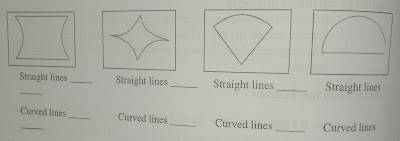# Lesson Plan of Geometry Elements

Lesson Planning of Geometry (Line and Curves)

Subject Mathematics

Students` Learning Outcomes

• Differentiate between a straight line and a curved line.
• Identify straight and curved lines from the given line drawings.
• Use straightedge / ruler to draw a straight line of given length (exclude fractional lengths)

Information for Teachers

• A line is a type of geometric figure. A line is made up of various numbers of points.
• A line can be straight or curved. A straight line shows the shortest distance between two points.
• Curved line distance is greater than the straight line. A curved line is sometimes called a curve, as;
• A circle shows curved lines whereas triangle, rectangle, and square show straight lines.
• We use a ruler to draw straight lines of given lengths.
• While teaching the lesson, the teacher should also consult textbook at all steps wherever and whenever it is applicable.

Material / Resources

Writing board, chalk/marker, duster, drawing having curved and straight lines on a card sheet, worksheet

Introduction

• Draw the shapes of square on the board and ask the students, are lines straight or curved? (The expected answer would be as; straight)
• Draw the shapes of circle on the board and ask the students, that line or curved. (The expected answer would be as; curved)

Development

 Activity 1 Draw some straight and curved line by drawing different lines on the board and ask which line is straight and which is curved. Draw some more lines (both straight and curved lines) on the board and ask the students to identify straight and curved lines, as;Activity 2 Provide the worksheet with different drawings on the board. Ask the students to identify the number of straight and curved lines in each line drawing and write in the spaces given.Activity 3 Demonstrate the drawing of a straight line using any straight edge (book, lunch box, pencil)

• Ask the students to draw straight lines on their notebooks using book, lunch box or pencil.
• Observe their work and guide them.
• Tell the students that we can draw straight line by using ruler.
• Ask the students to draw straight lines using ruler.
• They will compare these lines with the lines drawn by using books.

 Activity 4 Ask the students to do practice pages given on their Mathematics books.

Sum up /Conclusion

• A line can be straight or curved.
• We draw straight line using a ruler.
• We can draw a straight line of given length using a ruler.

Assessment

• Demonstrate the drawing of straight lines of the given lengths using a ruler. Ask the students to draw straight lines of the following lengths using a ruler.

a)                                      a)     8 cm

b)                                      b)   10 cm

c)                                       c)  6 cm

• Observe their work and guide. Encourage the students and help the slow learners.
• Teacher is also required to involve the students in solving the problems given in the exercise at the end of unit / chapter.

1.